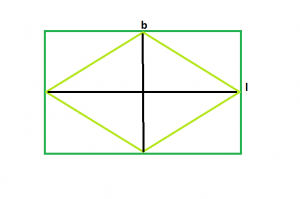# Area of the biggest possible rhombus that can be inscribed in a rectangle

• Last Updated : 29 Jun, 2022

Given a rectangle of length l and breadth b, the task is to find the largest rhombus that can be inscribed in the rectangle.
Examples

```Input : l = 5, b = 4
Output : 10

Input : l = 16, b = 6
Output : 48```From the figure, we can see, the biggest rhombus that could be inscribed within the rectangle will have its diagonals equal to the length & breadth of the rectangle.
So, Area of rhombus, A = (l*b)/2
Below is the implementation of the above approach:

## C++

 `// C++ Program to find the biggest rhombus` `// which can be inscribed within the rectangle` `#include ` `using` `namespace` `std;`   `// Function to find the area` `// of the biggest rhombus` `float` `rhombusarea(``float` `l, ``float` `b)` `{` `    ``// the length and breadth cannot be negative` `    ``if` `(l < 0 || b < 0)` `        ``return` `-1;`   `    ``// area of the rhombus` `    ``return` `(l * b) / 2;` `}`   `// Driver code` `int` `main()` `{` `    ``float` `l = 16, b = 6;` `    ``cout << rhombusarea(l, b) << endl;` `    ``return` `0;` `}`

## Java

 `// Java Program to find the ` `// biggest rhombus which can be ` `// inscribed within the rectangle` `import` `java.io.*;`   `class` `GFG ` `{`   `// Function to find the area` `// of the biggest rhombus` `static` `float` `rhombusarea(``float` `l,` `                         ``float` `b)` `{` `    ``// the length and breadth` `    ``// cannot be negative` `    ``if` `(l < ``0` `|| b < ``0``)` `        ``return` `-``1``;`   `    ``// area of the rhombus` `    ``return` `(l * b) / ``2``;` `}`   `// Driver code` `public` `static` `void` `main (String[] args) ` `{` `    ``float` `l = ``16``, b = ``6``;` `    ``System.out.println(rhombusarea(l, b));` `}` `}`   `// This code is contributed` `// by inder_verma`

## Python3

 `# Python 3 Program to find the biggest rhombus` `# which can be inscribed within the rectangle`     `# Function to find the area` `# of the biggest rhombus` `def` `rhombusarea(l,b):` `    ``# the length and breadth cannot be negative` `    ``if` `(l < ``0` `or` `b < ``0``):` `        ``return` `-``1`   `    ``# area of the rhombus` `    ``return` `(l ``*` `b) ``/` `2`   `# Driver code` `if` `__name__ ``=``=` `'__main__'``:` `    ``l ``=` `16` `    ``b ``=` `6` `    ``print``(rhombusarea(l, b))`

## C#

 `// C# Program to find the ` `// biggest rhombus which can be ` `// inscribed within the rectangle` `using` `System;`   `class` `GFG ` `{`   `// Function to find the area` `// of the biggest rhombus` `static` `float` `rhombusarea(``float` `l,` `                        ``float` `b)` `{` `    ``// the length and breadth` `    ``// cannot be negative` `    ``if` `(l < 0 || b < 0)` `        ``return` `-1;`   `    ``// area of the rhombus` `    ``return` `(l * b) / 2;` `}`   `// Driver code` `public` `static` `void` `Main () ` `{` `    ``float` `l = 16, b = 6;` `    ``Console.WriteLine(rhombusarea(l, b));` `}` `}`   `// This code is contributed` `// by shs`

## PHP

 `

## Javascript

 ``

Output:

`48`

Time complexity: O(1)

Auxiliary Space: O(1)

My Personal Notes arrow_drop_up
Recommended Articles
Page :